# NumPy Array – Logical Operations

• Difficulty Level : Easy
• Last Updated : 15 Mar, 2021

Logical operations are used to find the logical relation between two arrays or lists or variables. We can perform logical operations using NumPy between two data. Below are the various logical operations we can perform on Numpy arrays:

## AND

The numpy module supports the logical_and operator. It is used to relate between two variables. If two variables are 0 then output is 0, if two variables are 1 then output is 1 and if one variable is 0 and another is 1 then output is 0.

Syntax:

numpy.logical_and(var1,var2)

Where, var1 and var2 are a single variable or a list/array.

Return type: Boolean value (True or False)

Example 1:

This code gives demo on boolean operations with logical_and operator.

## Python3

 `# importing numpy module``import` `numpy as np`` ` `# list 1 represents an array with boolean values``list1 ``=` `[``True``, ``False``, ``True``, ``False``]`` ` `# list 2 represents an array with boolean values``list2 ``=` `[``True``, ``True``, ``False``, ``True``]`` ` `# logical operations between boolean values``print``(``'Operation between two lists =  '``,``      ``np.logical_and(list1, list2))`

Output: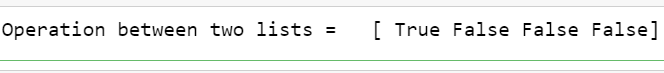Example 2:

## Python3

 `# importing numpy module``import` `numpy as np`` ` `# list 1 represents an array ``# with integer values``list1 ``=` `[``1``, ``2``, ``3``, ``4``, ``5``, ``0``]`` ` `# list 2 represents an array ``# with integer values``list2 ``=` `[``0``, ``1``, ``2``, ``3``, ``4``, ``0``]`` ` `# logical operations between integer values``print``(``'Operation between two lists:'``, ``      ``np.logical_and(list1, list2))`

Output: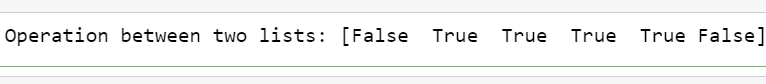## OR

The NumPy module supports the logical_or operator. It is also used to relate between two variables. If two variables are 0 then output is 0, if two variables are 1 then output is 1 and if one variable is 0 and another is 1 then output is 1.

Syntax:

numpy.logical_or(var1,var2)

Where, var1 and var2 are a single variable or a list/array.

Return type: Boolean value (True or False)

Example:

## Python3

 `# importing numpy module``import` `numpy as np`` ` `# logical operations between boolean values``print``(``'logical_or operation =  '``, ``      ``np.logical_or(``True``, ``False``))`` ` `a ``=` `2``b ``=` `6``print``(``'logical or Operation between two variables =  '``, ``      ``np.logical_or(a, b))``a ``=` `0``b ``=` `0``print``(``'logical or Operation between two variables =  '``, ``      ``np.logical_or(a, b))`` ` ` ` `# list 1 represents an array with integer values``list1 ``=` `[``1``, ``2``, ``3``, ``4``, ``5``, ``0``]`` ` `# list 2 represents an array with integer values``list2 ``=` `[``0``, ``1``, ``2``, ``3``, ``4``, ``0``]`` ` `# logical operations between integer values``print``(``'Operation between two lists =  '``, ``      ``np.logical_or(list1, list2))`

Output: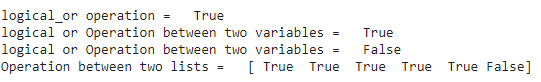## NOT

The logical_not operation takes one value and converts it into another value. If the value is 0, then output is 1, if value is greater than or equal to 1 output is 0.

Syntax:

numpy.logical_not(var1)

Where, var1is a single variable or a list/array.

Return type: Boolean value (True or False)

Example:

## Python3

 `# importing numpy module``import` `numpy as np`` ` `# logical  not operations for boolean value``print``(``'logical_not operation =  '``, ``      ``np.logical_not(``True``))`` ` `a ``=` `2``b ``=` `6``print``(``'logical_not Operation  =  '``, ``      ``np.logical_not(a))``print``(``'logical_not Operation  =  '``, ``      ``np.logical_not(b))`` ` ` ` `# list 1 represents an array with integer values``list1 ``=` `[``1``, ``2``, ``3``, ``4``, ``5``, ``0``]`` ` `# logical operations between integer values``print``(``'Operation in list =  '``, ``      ``np.logical_not(list1))`

Output: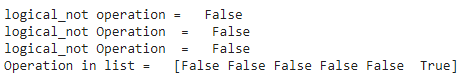## XOR

The logical_xor performs the xor operation between two variables or lists. In this operation, if two values are same it returns 0 otherwise 1.

Syntax:

numpy.logical_xor(var1,var2)

Where, var1 and var2 are a single variable or a list/array.

Return type: Boolean value (True or False)

Example:

## Python3

 `# importing numpy module``import` `numpy as np`` ` `# logical operations between boolean values``print``(``'Operation between true and true ( 1 and 1) =  '``, ``      ``np.logical_xor(``True``, ``True``))``print``(``'Operation between true and false ( 1 and 0) = '``,``      ``np.logical_xor(``True``, ``False``))``print``(``'Operation between false and true ( 0 and 1) = '``,``      ``np.logical_xor(``False``, ``True``))``print``(``'Operation between false and false (0 and 0)= '``,``      ``np.logical_xor(``False``, ``False``))`` ` ` ` `# list 1 represents an array with boolean values``list1 ``=` `[``True``, ``False``, ``True``, ``False``]`` ` `# list 2 represents an array with boolean values``list2 ``=` `[``True``, ``True``, ``False``, ``True``]`` ` `# logical operations between boolean values``print``(``'Operation between two lists =  '``, ``      ``np.logical_xor(list1, list2))`` ` ` ` `# list 1 represents an array ``# with integer values``list1 ``=` `[``1``, ``2``, ``3``, ``4``, ``5``, ``0``]`` ` `# list 2 represents an array ``# with integer values``list2 ``=` `[``0``, ``1``, ``2``, ``3``, ``4``, ``0``]`` ` `# logical operations between integer values``print``(``'Operation between two lists =  '``, ``      ``np.logical_xor(list1, list2))`

Output: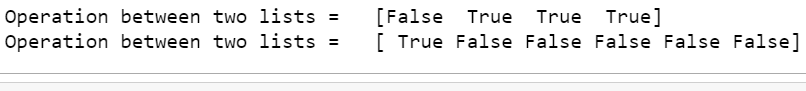My Personal Notes arrow_drop_up